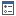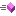﻿ Envelope Class Members—ArcGIS Pro
ArcGIS Pro 2.6 API Reference Guide
Envelope Class Members
Properties  Methods

 ArcGIS.Core.Geometry Namespace : Envelope Class

The following tables list the members exposed by Envelope.

Public Properties
NameDescriptionGets the area of this instance.Gets the center of this instance as a MapPoint.Gets the center of this instance as a Coordinate2D.Gets the depth of this instance.Gets the dimension of the geometry. (Inherited from ArcGIS.Core.Geometry.Geometry)Overridden.  Gets the minimum enclosing envelope of this instance. Returns null if this geometry has IsEmpty = true.Overridden. Gets the GeometryType of this instance. Always returns GeometryType.Envelope.Gets a value indicating if the geometry has ID. (Inherited from ArcGIS.Core.Geometry.Geometry)Gets a value indicating if the geometry has M. (Inherited from ArcGIS.Core.Geometry.Geometry)Gets a value indicating if the geometry has Z. (Inherited from ArcGIS.Core.Geometry.Geometry)Gets the height of this instance.Overridden.  Gets a value indicating whether or not the geometry is empty.Gets the perimeter length of this instance.Gets the 3D length of the perimeter of this instance.Gets the M maximum of this instance.Gets the M minimum of this instance.Overridden.  Gets the point count of this instance which is always 4.Gets the spatial reference of the instance. (Inherited from ArcGIS.Core.Geometry.Geometry)Gets the width of this instance.Gets the X maximum of this instance.Gets the X minimum of this instance.Gets the Y maximum of this instance.Gets the Y minimum of this instance.Gets the Z maximum of this instance.Gets the Z minimum of this instance.
Top
Public Methods
NameDescriptionCenter the envelope at the specified x and y coordinate.Clones this geometry instance. As geometry objects are immutable and hence never change, the clone returned will be this object rather than a copy of this instance. (Inherited from ArcGIS.Core.Geometry.Geometry)Creates a new envelope expanded by the amount specified. Expand moves the X and Y coordinates of the sides toward or away from each other to scale the size of the Envelope.Calculates the intersection between this instance and the specified envelope.Determines if this instance intersects the supplied envelope.Overloaded.Overloaded. Overridden.Serializes this geometry instance into an ArcGIS JSON geometry representation. (Inherited from ArcGIS.Core.Geometry.Geometry)Serializes this geometry instance into an ArcGIS XML geometry representation. (Inherited from ArcGIS.Core.Geometry.Geometry)Returns the union of this instance and the specified envelope.
Top